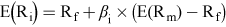### Become an OU studentStart this free course now. Just create an account and sign in. Enrol and complete the course for a free statement of participation or digital badge if available.

# 3.6 Using the CAPM to find a project-specific cost of equity

In order to adjust for a difference in business risk between the company and a new project, it is possible to use the capital asset pricing model (CAPM) to calculate the return on equity that would be required for the new project. Then this figure can be used to calculate an effective WACC for the project. Doing this means the project is being treated as if it were an independent business, and the price of equity would be worked out as if it were a company whose only operation was the project under consideration.

Using the CAPM allows you to appraise a project on the basis of the risk of that project specifically, rather than the risks that apply to the company as a whole. This allows decisions about whether to proceed with a project to be more accurately focused. Another benefit is that projects with different risk profiles can be compared with each other. Using the same discount rate to appraise different projects is misleading, as it implies that all projects have the same risk profile, whereas riskier projects should have their cash flows discounted to a greater extent to reflect this.

To calculate the cost of equity for a project, it is necessary to find a group of companies which operate in the industry the new project will be in. Beta values are published and available publicly, so the average beta value for companies in the industry can be calculated and used to work out the equity cost of capital.

Remember, the formula for calculating the cost of equity is:Equation 8

where:

E(Ri) = the expected return on shares of company i = cost of equity

Rf = the risk-free rate of return

E(Rm) = the expected return on the market

E(Rm) – Rf = the expected equity risk premium

βi = the beta for the company

## Activity 6 Using NPV to choose between projects

Timing: Spend about 15 minutes on this activity.

A company wants to choose between two projects. Find the net present value of each project, based on the company’s cost of capital which is 10%. Assume that the company is entirely financed by equity. Make a recommendation about which project should be accepted.

Table 13
Cash flow (£)Year 0Year 1Year 2Year 3
Project A(70,000)30,00030,00030,000
Project B(25,000)10,00010,00010,000

Table 14 Project A
Year of cash flow0123Total
Cash flow (£)(70,000)30,00030,00030,000
Discount factor of 10%1.00000.90910.82640.7513
Discounted cash flow(70,000)27,27324,79222,5394,604
Table 15 Project B
Year of cash flow0123Total
Cash flow (£)(25,000)10,00010,00010,000
Discount factor of 10%1.00000.90910.82640.7513
Discounted cash flow(25,000)9,0918,2647,513(132)

The discounted cash flow of Project A is £4,604, compared with (£132) for Project B. Therefore Project A should be accepted.

## Activity 7 Using the CAPM to calculate a discount rate

Timing: Spend about 25 minutes on this activity.

The company in Activity 6 is still choosing between Projects A and B. However, there is now some additional information.

The beta value of the company is 0.875. Assume a risk-free rate of 3% and expected equity risk premium of 8%. The average beta value of equity capital for companies in the industry relevant to Project A is 1.5 and the average beta value of equity capital for companies in the industry relevant to Project B is 0.375.

### Question 1

1 Calculate the expected return (cost of equity) using CAPM for Projects A and B.

Project A

The average expected return for this industry is 3.0% + (1.5 × 8%) = 15% p. a.

Project B

The average expected return for this industry is 3.0% + (0.375 × 8%) = 6% p. a.

Note that the expected return, or cost of capital, for the company’s current industry is 3.0% + (0.875 × 8%) = 10% p.a. However, since the business risk in the company’s current industry is different from the industries relevant to Projects A and B, knowing the expected return for the company’s current industry is not relevant. Indeed, you should be able to see that using 10% as the expected return to assess the new projects is not appropriate, given they both differ from this expected return.

### Question 2

2 Find the net present value of each project, based on the cost of equity for each project implied by CAPM. Again assume that the company is entirely financed by equity. Make a recommendation about which project should be accepted.